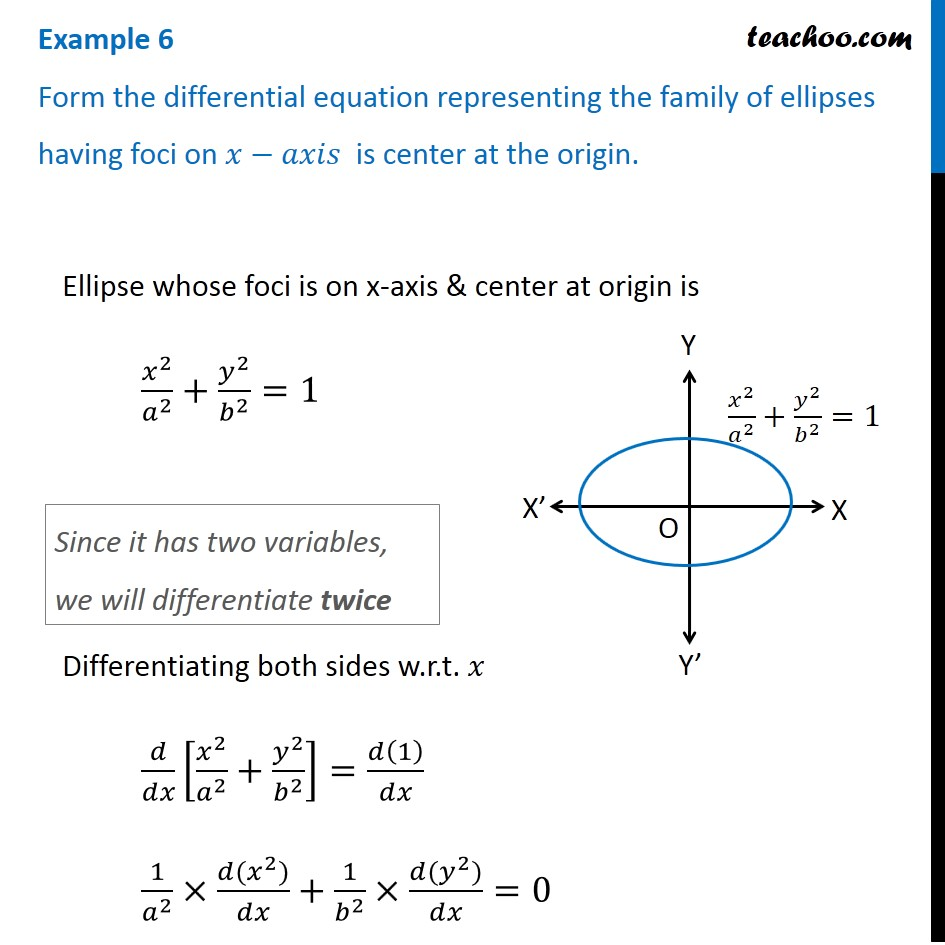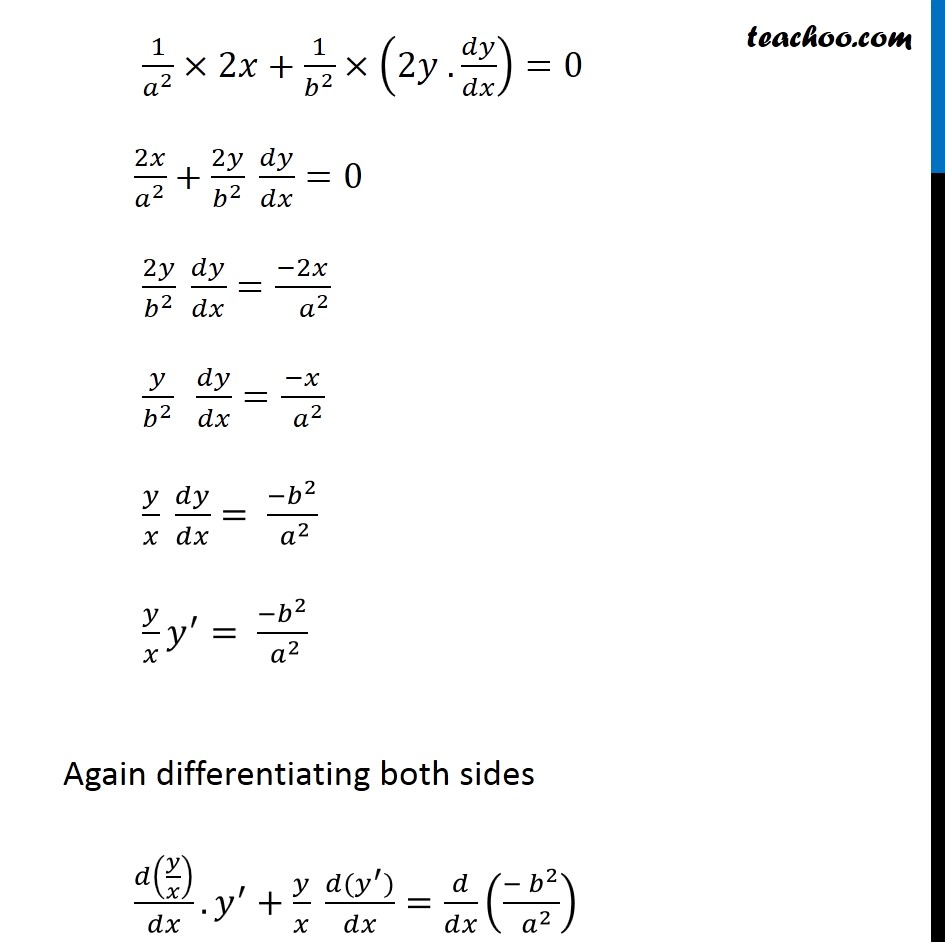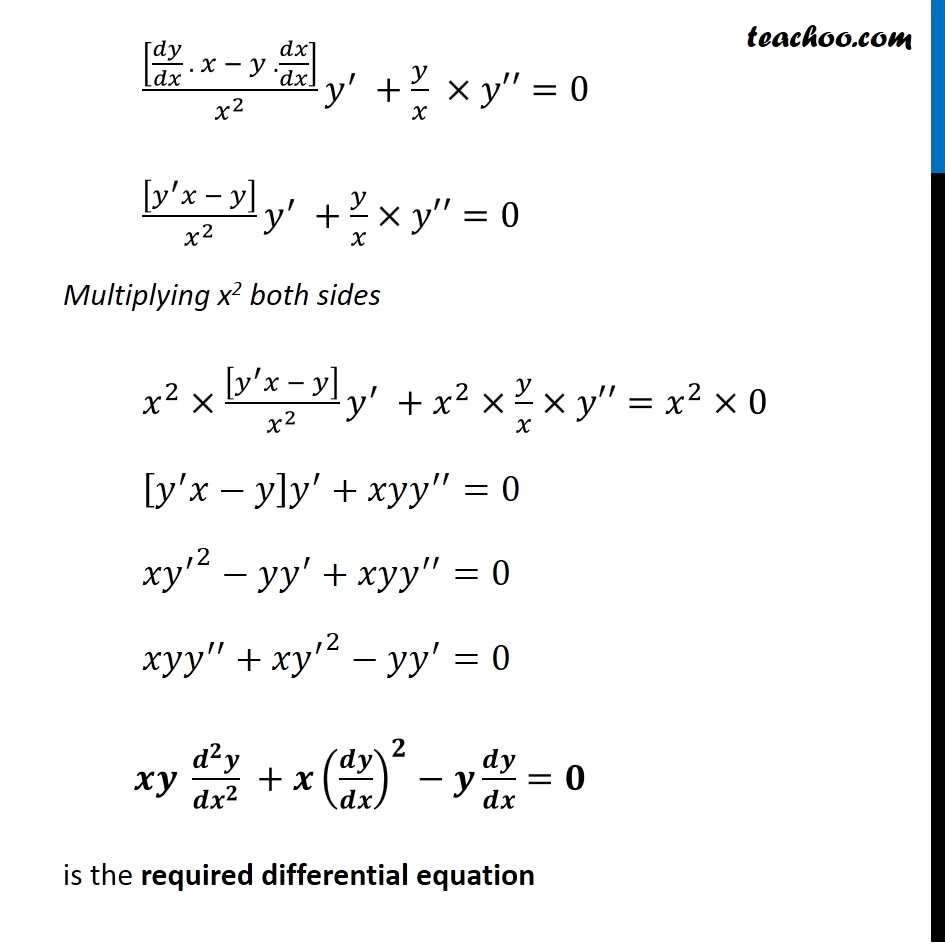Examples

Chapter 9 Class 12 Differential Equations
Serial order wiseLearn in your speed, with individual attention - Teachoo Maths 1-on-1 Class

### Transcript

Question 3 Form the differential equation representing the family of ellipses having foci on 𝑥−𝑎𝑥𝑖𝑠 is center at the origin. Ellipse whose foci is on x-axis & center at origin is 𝑥^2/𝑎^2 +𝑦^2/𝑏^2 =1 Differentiating both sides w.r.t. 𝑥 𝑑/𝑑𝑥 [𝑥^2/𝑎^2 +𝑦^2/𝑏^2 ]=𝑑(1)/𝑑𝑥 1/𝑎^2 ×(〖𝑑(𝑥〗^2))/𝑑𝑥+1/𝑏^2 ×(〖𝑑(𝑦〗^2))/𝑑𝑥=0 Since it has two variables, we will differentiate twice 𝑥^2/𝑎^2 +𝑦^2/𝑏^2 =1 1/𝑎^2 ×2𝑥+1/𝑏^2 ×(2𝑦 . 𝑑𝑦/𝑑𝑥)=0 2𝑥/𝑎^2 +2𝑦/𝑏^2 𝑑𝑦/𝑑𝑥=0 2𝑦/𝑏^2 𝑑𝑦/𝑑𝑥=(−2𝑥)/〖 𝑎〗^2 𝑦/𝑏^2 𝑑𝑦/𝑑𝑥=(−𝑥)/〖 𝑎〗^2 𝑦/𝑥 𝑑𝑦/𝑑𝑥= (−𝑏^2)/〖 𝑎〗^2 𝑦/𝑥 𝑦^′= (−𝑏^2)/〖 𝑎〗^2 Again differentiating both sides 𝑑(𝑦/𝑥)/𝑑𝑥. 𝑦^′+𝑦/𝑥 (𝑑(𝑦^′))/𝑑𝑥=𝑑/𝑑𝑥 ((− 𝑏^2)/( 𝑎^2 )) [𝑑𝑦/𝑑𝑥 . 𝑥 − 𝑦 .𝑑𝑥/𝑑𝑥]/𝑥^2 𝑦^′ +𝑦/𝑥 ×𝑦′′=0 [𝑦^′ 𝑥 − 𝑦]/𝑥^2 𝑦^′ +𝑦/𝑥×𝑦′′=0 Multiplying x2 both sides 𝑥^2×[𝑦^′ 𝑥 − 𝑦]/𝑥^2 𝑦^′ +𝑥^2×𝑦/𝑥×𝑦′′=𝑥^2×0 [𝑦^′ 𝑥−𝑦] 𝑦^′+𝑥𝑦𝑦^′′=0 〖〖𝑥𝑦〗^′〗^2−𝑦𝑦^′+𝑥𝑦𝑦^′′=0 𝑥𝑦𝑦^′′+〖〖𝑥𝑦〗^′〗^2−𝑦𝑦^′=0 𝒙𝒚 (𝒅^𝟐 𝒚)/(𝒅𝒙^𝟐 ) +𝒙(𝒅𝒚/𝒅𝒙)^𝟐−𝒚 𝒅𝒚/𝒅𝒙=𝟎 is the required differential equation